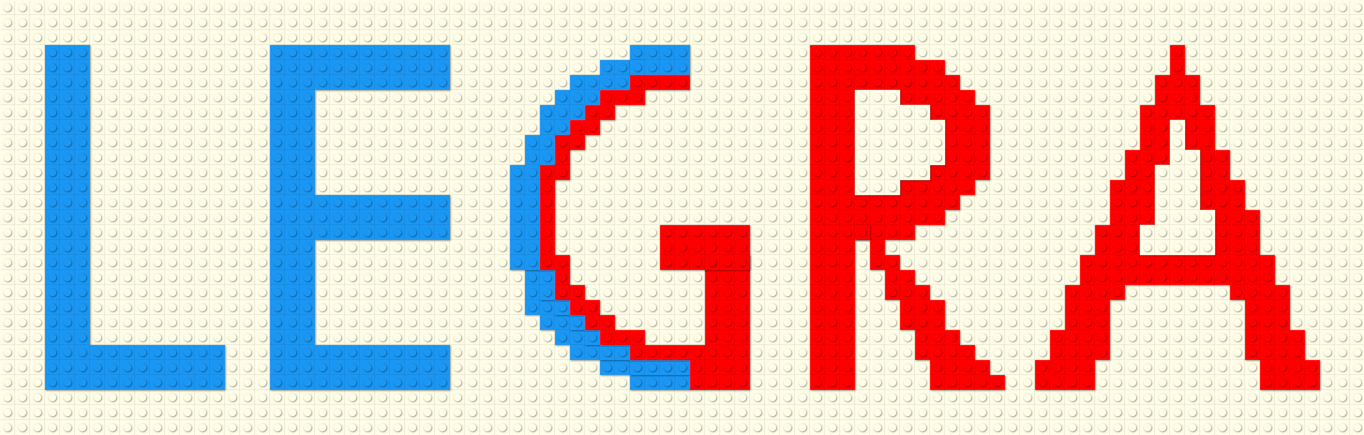# Legra

Legra (Lego brick Graphics) is a small (3.36KB gzipped) JavaScript library that lets you draw using LEGO® like brick shapes on an HTML `<canvas>` element. This library defines basic graphics primitives like lines, rectangles, polygons, ellipses, bézier curves, etc. All shapes are drawn either outlined or filled in.

The size and color of the bricks used can be configured. Legra can be used to create fun digital sketches, diagrams, or data visualizations.

View on Github

## Examples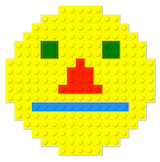Animated smiley face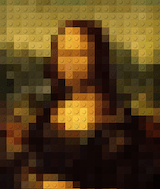Mona Lisa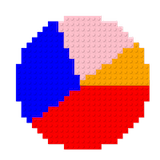Simple pie chart

All examples with source are available on Legra Glitch collection

## Install

Install from npm

``npm install --save legra``

Or source it directly in your web page:

``<script src="https://unpkg.com/legra?browser"></script>``

## Usage

Instantiate legra instance with your `<canvas>`'s 2D context.

The constructor, optionally, takes in two other arguments - The brick size, which defaults to 24px, and `BrickRenderOptions` object where you can specify the default brick color.

``import Legra from 'legra'const ctx = myCanvas.getContext('2d');const legra = new Legra(ctx);// orconst legra = new Legra(ctx, 18);// orconst legra = new Legra(ctx, 32, {color: 'blue'});``

Now you can call the following drawing methods. Each method takes in an optional `BrickRenderOptions` object where you can specify the color of the shape, and if the shape should be filled in.

Note: All lengths are specified in brick units, and not pixels. e.g. width of 5 means 5 bricks wide.

## Line

Draws a line from (x1, y1) to (x2, y2).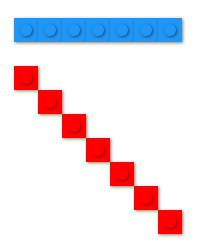``// line(x1, y1, x2, y2 [, options])legra.line(3, 1, 10, 1);legra.line(11, 11, 1, 1, { color: 'red' });``

## Rectangle

Draws a rectangle with the top-left corner at (x, y) with the specified width and height.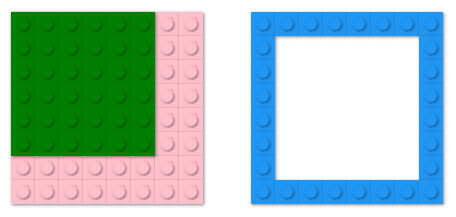``// rectangle(x, y, width, height [, options])legra.rectangle(12, 2, 8, 8);legra.rectangle(2, 2, 8, 8, { filled: true, color: 'pink' });legra.rectangle(2, 2, 6, 6, { filled: true, color: 'green' });``

## Linear path

Draws a set of lines connecting the specified points. `points` is an array of points. Each point is an array with 2 values - `[x, y]`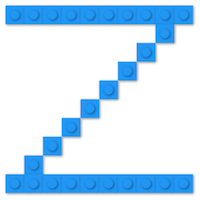``// linearPath(points [, options])legra.linearPath([  [1, 1],  [10, 1],  [1, 10],  [10, 10]]);``

## Ellipse and Circle

Draws an ellipse/circle with the center at `(xc, yc)`. For the ellipse, `a` and `b` are the horizontal and vertical semi-axis lengths respectively.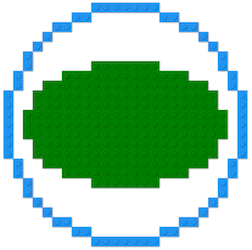``// circle(xc, yc, radius [, options])// ellipse(xc, yc, a, b, [, options])legra.circle(15, 15, 12);legra.ellipse(15, 15, 10, 6, { filled: true, color: 'green' });``

## Polygon

Draws a polygon with the specified vertices. `vertices` is an array of points. Each point is an array with 2 values - `[x, y]`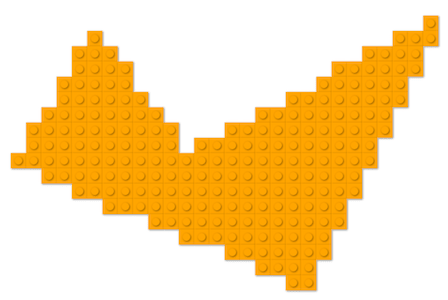``// polygon(vertices [, options])legra.polygon([  [3, 10],  [22, 18],  [30, 1],  [14, 10],  [8, 2]], { filled: true, color: 'orange' });``

## Arc

Draws an arc. An arc is described as a section of en ellipse. `x`, `y` represent the center of that ellipse. `a` and `b` are the horizontal and vertical semi-axis lengths, respectively, for that ellipse.

`start`, `stop` are the start and stop angles for the arc.

`closed` is a boolean argument. If true, lines are drawn to connect the two end points of the arc to the center.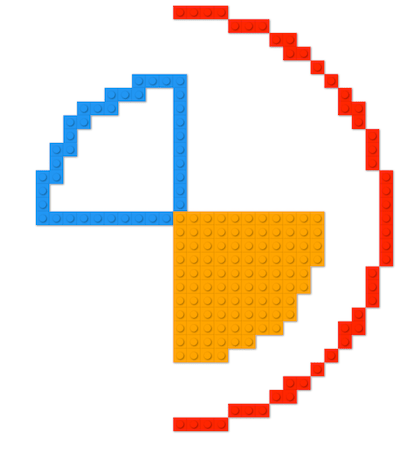``// arc(xc, yc, a, b, start, stop, closed [, options])legra.arc(15, 15, 10, 10, Math.PI, Math.PI * 1.5, true);legra.arc(15, 15, 10, 10, 0, Math.PI * .5, true, {   filled: true,   color: 'orange' });legra.arc(15, 15, 15, 15, -Math.PI * 0.5, Math.PI * 0.5, false, {   color: 'red' });``

## Bézier curve

Draws a bézier curve from `(x1, y1)` to `(x2, y2)` with `(cp1x, cp1y)` and `(cp2x, cp2y)` as the curve's control points.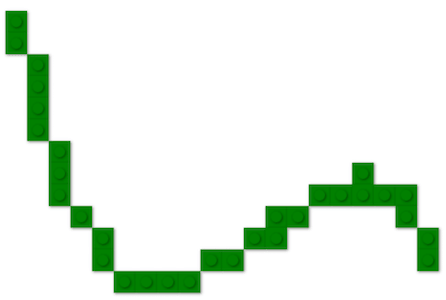``// bezierCurve(x1, y1, cp1x, cp1y, cp2x, cp2y, x2, y2 [, options])legra.bezierCurve(3, 3, 8, 30, 18, 1, 22, 14);``

Draws a quadratic curve from `(x1, y1)` to `(x2, y2)` with `(cpx, cpy)` as the curve's control point.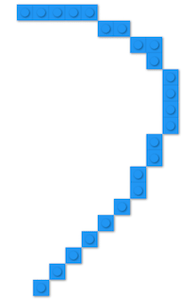``// quadraticCurve(x1, y1, cpx, cpy, x2, y2 [, options])legra.quadraticCurve(3, 3, 8, 30, 18, 1, 22, 14);``

## Draw image

Draws an image at point `dst`. Optionally, one can provide the size of the image to be drawn, and the source area to be drawn. Points and Sizes are defined as an array of two number `[x, y]` and `[width, height]` respectively.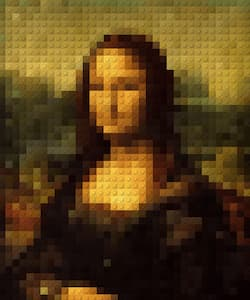``// drawImage(image, dstPoint [, dstSize, srcPoint, srcSize])legra.drawImage(image, [0, 0]);``

## Future work

The current version of Legra is not optimized for large canvases. Legra can be used with an OffscreenCanvas in a worker thread to improve perceived performance.

A future version should use a WebGL shader for rendering.

## Credits

Code to calculate Bézier curve points has been adapted from bezier.js.

Algorithm to fill ellipses has been adopted from this paper: Drawing Ellipses Using Filled Rectangles.